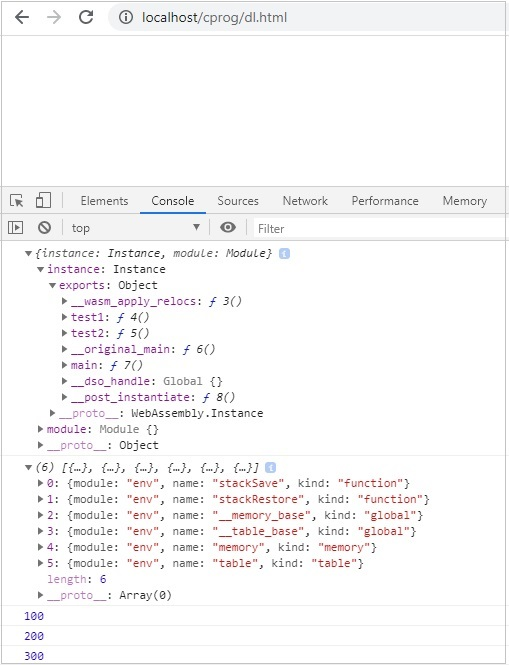Dynamic linking is the process in which two or more modules will be linked together during runtime.

To demonstrate how dynamic linking works, we will use C program and compile it to wasm using Ecmascript sdk.

So here we have −

test1.c

```int test1(){
return 100;
}
```

test2.c

```int test2(){
return 200;
}
```

main.c

```#include <stdio.h>

int test1();
int test2();
int main() {
int result = test1() + test2();
return result;
}
```

In main.c code, it makes use of test1() and test2(), which are defined inside test1.c and test2.c. Let us check how to link these modules in WebAssembly.

The command to compile the above code is as follows: make use of SIDE_MODULE =1 for dynamic linking as shown in the command.

```emcc test1.c test2.c main.c -s SIDE_MODULE=1 -o maintest.wasm
```

Using WasmtoWat, which is available at https://webassembly.github.io/wabt/demo/wasm2wat/, will get the WebAssembly text format of maintest.wasm.

```(module
(type \$t0 (func (result i32))) (type \$t1 (func))
(type \$t2 (func (param i32))) (type \$t3 (func (param i32 i32) (result i32)))
(import "env" "stackSave" (func \$env.stackSave (type \$t0)))
(import "env" "stackRestore" (func \$env.stackRestore (type \$t2)))
(import "env" "__memory_base" (global \$env.__memory_base i32))
(import "env" "__table_base" (global \$env.__table_base i32))
(import "env" "memory" (memory \$env.memory 0))
(import "env" "table" (table \$env.table 0 funcref))
(func \$f2 (type \$t1)
(call \$__wasm_apply_relocs)
)
(func \$__wasm_apply_relocs (export "__wasm_apply_relocs") (type \$t1))
(func \$test1 (export "test1") (type \$t0) (result i32)
(local \$l0 i32)
(local.set \$l0
(i32.const 100)
)
(return
(local.get \$l0)
)
)
(func \$test2 (export "test2") (type \$t0) (result i32)
(local \$l0 i32)
(local.set \$l0
(i32.const 200))
(return
(local.get \$l0)
)
)
(func \$__original_main
(export "__original_main")
(type \$t0)
(result i32)
(local \$l0 i32)
(local \$l1 i32)
(local \$l2 i32)
(local \$l3 i32)
(local \$l4 i32)
(local \$l5 i32)
(local \$l6 i32)
(local \$l7 i32)
(local \$l8 i32)
(local \$l9 i32)
(local.set \$l0(call \$env.stackSave))
(local.set \$l1 (i32.const 16))
(local.set \$l2 (i32.sub (local.get \$l0) (local.get \$l1)))
(call \$env.stackRestore (local.get \$l2) ) (local.set \$l3(i32.const 0))
(i32.store offset=12 (local.get \$l2) (local.get \$l3))
(local.set \$l4 (call \$test1))
(local.set \$l5 (call \$test2))
(local.set \$l6 (i32.add (local.get \$l4) (local.get \$l5)))
(i32.store offset=8 (local.get \$l2) (local.get \$l6))
(local.set \$l7 (i32.load offset=8 (local.get \$l2)))
(local.set \$l8 (i32.const 16))
(local.set \$l9 (i32.add (local.get \$l2) (local.get \$l8)))
(call \$env.stackRestore (local.get \$l9)) (return(local.get \$l7))
)
(func \$main
(export "main")
(type \$t3)
(param \$p0 i32)
(param \$p1 i32)
(result i32)
(local \$l2 i32)
(local.set \$l2
(call \$__original_main))
(return (local.get \$l2))
)
(func \$__post_instantiate (export "__post_instantiate") (type \$t1) (call \$f2))
(global \$__dso_handle (export "__dso_handle") i32 (i32.const 0))
)
```

The WebAssembly text format has some imports defined as shown below −

```(import "env" "stackSave" (func \$env.stackSave (type \$t0)))
(import "env" "stackRestore" (func \$env.stackRestore (type \$t2)))
(import "env" "__memory_base" (global \$env.__memory_base i32))
(import "env" "__table_base" (global \$env.__table_base i32))
(import "env" "memory" (memory \$env.memory 0))
(import "env" "table" (table \$env.table 0 funcref))
```

This is added while compiling code by emcc(emscripten sdk) and it deals with memory management in WebAssembly.

## Working with Imports and Exports

Now to see the output, we will have to define the imports that you can see in the .wat code −

```(import "env" "stackSave" (func \$env.stackSave (type \$t0)))
(import "env" "stackRestore" (func \$env.stackRestore (type \$t2)))
(import "env" "__memory_base" (global \$env.__memory_base i32))
(import "env" "__table_base" (global \$env.__table_base i32))
(import "env" "memory" (memory \$env.memory 0))
(import "env" "table" (table \$env.table 0 funcref))
```

The above terms are explained as follows −

• env.stackSave − It is used for stack management, a functionality that is provided by the emscripten compiled code.

• env.stackRestore − It is used for stack management, a functionality that is provided by the emscripten compiled code.

• env.__memory_base − It is an immutable i32 global offset that is, used in env.memory and reserved for the wasm module. The module can use this global in the initializer of its data segments, so that, they are loaded at the correct address.

• env.__table_base − It is an immutable i32 global offset that is, used in env.table and reserved for the wasm module. The module can use this global in the initializer of its table element segments, so that, they are loaded at the correct offset.

• env.memory − This will have the memory details that are required to be shared between the wasm modules.

• env.table − This will have the table details that are required to be shared between the wasm modules.

The imports have to be defined in javascript as follows −

```var wasmMemory = new WebAssembly.Memory({'initial': 256,'maximum': 65536});
const importObj = {
env: {
stackSave: n => 2, stackRestore: n => 3, //abortStackOverflow: () => {
throw new Error('overflow');
},
table: new WebAssembly.Table({
initial: 0, maximum: 65536, element: 'anyfunc'
}), __table_base: 0,
memory: wasmMemory, __memory_base: 256
}
};
```

### Example

Following is the javascript code that makes use of the importObj inside WebAssembly.instantiate.

```<!DOCTYPE html>
<html>
<meta charset="UTF-8">
<body>
<script>
var wasmMemory = new WebAssembly.Memory({'initial': 256,'maximum': 65536});
const importObj = {
env: {
stackSave: n => 2, stackRestore: n => 3, //abortStackOverflow: () => {
throw new Error('overflow');
},
table: new WebAssembly.Table({
initial: 0, maximum: 65536, element: 'anyfunc'
}), __table_base: 0,
memory: wasmMemory, __memory_base: 256
}
};
fetch("maintest.wasm") .then(bytes => bytes.arrayBuffer()) .then(
module => WebAssembly.instantiate(module, importObj)
)
.then(finalcode => {
console.log(finalcode);
console.log(WebAssembly.Module.imports(finalcode.module));
console.log(finalcode.instance.exports.test1());
console.log(finalcode.instance.exports.test2());
console.log(finalcode.instance.exports.main());
});
</script>
</body>
</html>
```

### Output

The output is as follows −